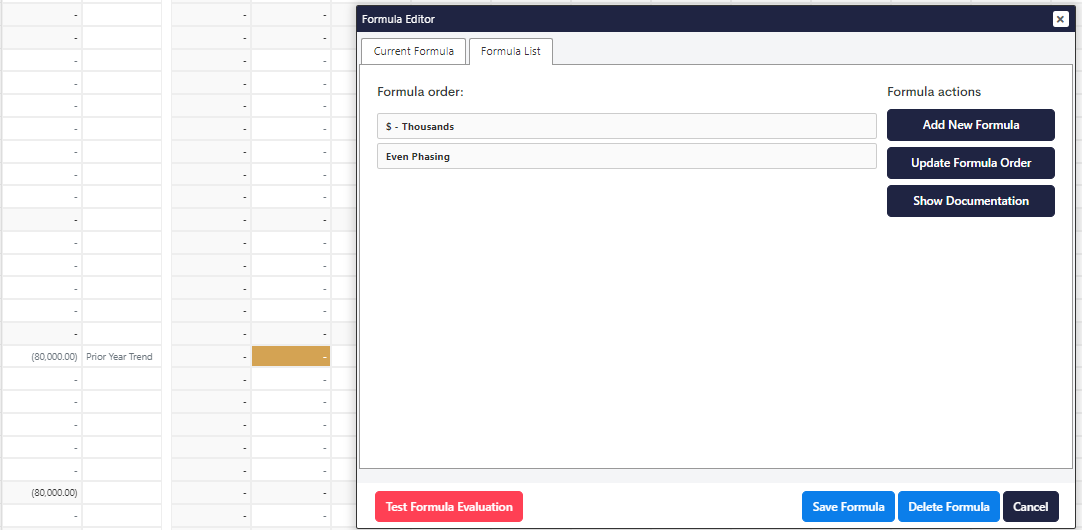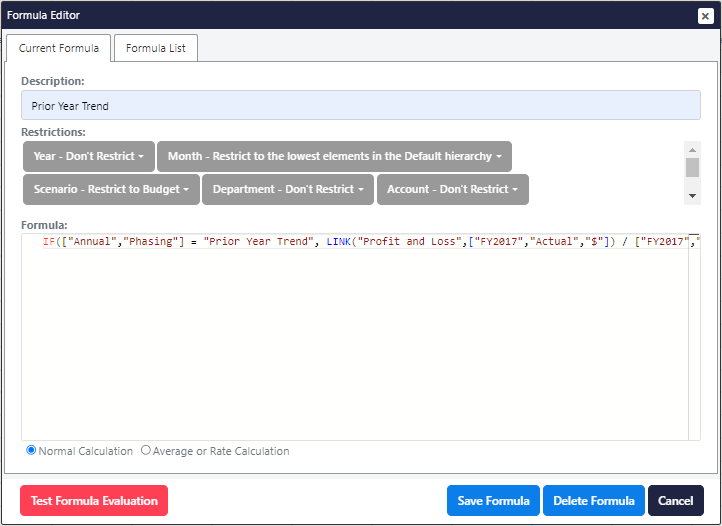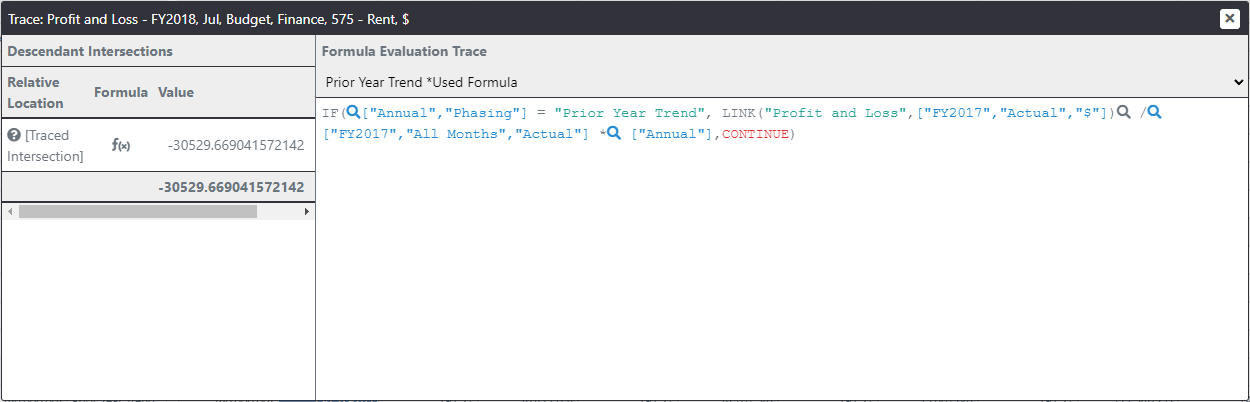# Prior Year Trend formula ​

Presently if we set the Phasing to Prior Year Trend no formula is applied and so I can change the value of the cell.

This formula is a bit more complicated. We could simplify it by writing a specific formula for each month but that would be a bit inefficient.

Instead, we will use the LINK function which can refer to a relative cell within this or any other cube.

Note: If when you right click to edit the formula you see the Even Phasing formula instead of seeing an empty formula, go to Formula List and click on Add New Formula.This rule begins in a similar way, first, we restrict the cells which the formula applies to as shown in the screenshot.

js
``IF(["Annual", "Phasing"] = "Prior Year Trend", LINK("Profit and Loss", ["FY2017", "Actual", "\$"]) / ["FY2017", "All Months", "Actual"] * ["Annual"], CONTINUE)``
``IF(["Annual", "Phasing"] = "Prior Year Trend", LINK("Profit and Loss", ["FY2017", "Actual", "\$"]) / ["FY2017", "All Months", "Actual"] * ["Annual"], CONTINUE)``The formula will check that the phasing method is indeed Prior Year Trend in which case it will take the same month from the prior years actual.

This link function sources information from the specified cube at the specified location, any dimensions which are not represented in the location argument will refer to the target cells relative location.

The resulting value will be used as a portion of the prior year's total value and multiply that by our phasing amount.

As I save that we can see that the annual budget matches our phasing amount but now the months are populated using the relativity from the prior year's actuals.

We can change the phasing method and our plan will recalculate in real-time.

If we explain the calculated cell, we can see that it depends upon the values in each month of the prior year, it works out the given month's portion of last years actuals and multiplies that by our phasing amount.Last updated: